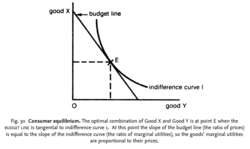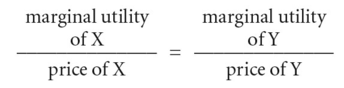# consumer equilibriumFig. 30 Consumer equilibrium. The optimal combination of Good X and Good Y is at point E when the BUDGET LINE is tangential to indifference curve 1. At this point the slope of the budget line (the ratio of prices)

## consumer equilibrium

the point at which the consumer maximizes his TOTAL UTILITY or satisfaction from the spending of a limited (fixed) income. The economic ‘problem’ of the consumer is that he has only a limited amount of income to spend and therefore cannot buy all the goods and services he would like to have. Faced with this constraint, demand theory assumes that the goal of the consumer is to select that combination of goods, in line with his preferences, that will maximize his total utility or satisfaction. Total utility is maximized when the MARGINAL UTILITY of a penny's worth of good X is exactly equal to the marginal utility of a penny's worth of all the other goods purchased; or, restated, when the prices of goods are different, the marginal utilities are proportional to their respective prices. For two goods, X and Y, total utility is maximized when:Consumer equilibrium can also be depicted graphically using INDIFFERENCE CURVE analysis. See Fig. 30 . See also REVEALED PREFERENCE THEORY,PRICE EFFECT, INCOME EFFECT, SUBSTITUTION EFFECT, ECONOMIC MAN, CONSUMER RATIONALITY, PARETO OPTIMALITY is equal to the slope of the indifference curve (the ratio of marginal utilities), so the goods’ marginal utilities are proportional to their prices.

Collins Dictionary of Economics, 4th ed. © C. Pass, B. Lowes, L. Davies 2005
References in periodicals archive ?
and the consumer equilibrium quantities of the two goods for an agent of type i [member of] {[alpha], [beta]} are
The market stationary equilibria, at which for every t the population shares and thus also the market equilibrium price and the consumer equilibrium quantities are constant, will be called trivial if they are not characterized by the coexistence between the two groups of agents, and nontrivial otherwise.
(IRI) has found a new consumer equilibrium emerging as the economy transforms.
"Since the beginning of 2009, a new consumer equilibrium has emerged in which behaviors first implemented to weather a storm have the potential to last well beyond an economic recovery," says IRI consulting and innovation president Thom Blischok.
This section derives the condition for consumer equilibrium. The condition is derived through sequential use of the definitions of MRS and RP.
The present analysis differs from utility-based demand theory in having its consumer equilibrium condition in the form of a single equation:
Denoting the reservation price by [r.sup.k] for k = h, l, a consumer equilibrium is generated by (8) [Mathematical Expression Omitted] for i, j = d, f; i [is not equal to] j and [p.sub.i] > [p.sub.j].
The present analysis differs from utility-based demand theory by having its consumer equilibrium condition in the form of a single equation:
This paper has shown that consumer equilibrium can be thought of as achieved sequentially and that the consumer chooses the amount of each good for which its MRS equals its RP.

Site: Follow: Share:
Open / Close